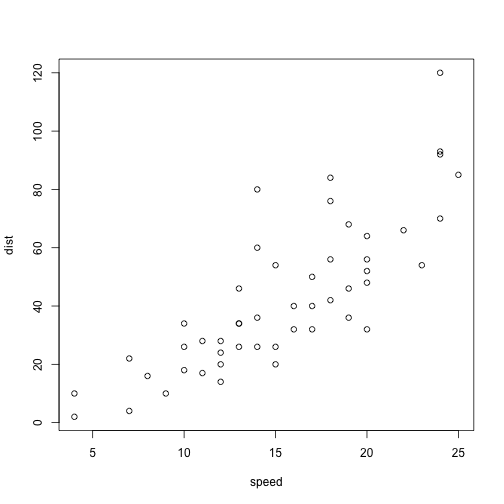# States Geo?

## R Markdown

``````library(deSolve)
library(ggplot2)
library(dplyr)
library(data.table)
library(tidyr)
library(lubridate)
library(plotly)
library(zoo)
library(cronR)
library(rvest)
library(httr)
library(XML)
library(jsonlite)
library(dplyr)
library(purrr)
library(tibble)
library(foreach)
library(doParallel)

cases_av_1000<-(ox_US_cur\$NewCases_07da/ox_US_cur\$total_pop)*1000

f4 <- list(size = 30,
color = "black")

g <- list(
scope = 'usa',
projection = list(type = 'albers usa'),
lakecolor = toRGB('white')
)

plot_geo() %>%
z = cases_av_1000, text = state.name,
locations = state.abb, locationmode = 'USA-states', colors= "Oranges", color = I("gray95")
) %>% layout(geo = g, title = list(text='US COVID-19 Cases 7-day Avg/1000 people', font=f4), margin = list(t = 100))
``````
```## Error in loadNamespace(name): there is no package called 'webshot'
```

This is an R Markdown document. Markdown is a simple formatting syntax for authoring HTML, PDF, and MS Word documents. For more details on using R Markdown see http://rmarkdown.rstudio.com.

When you click the Knit button a document will be generated that includes both content as well as the output of any embedded R code chunks within the document. You can embed an R code chunk like this:

``````summary(cars)
``````
```##      speed           dist
##  Min.   : 4.0   Min.   :  2.00
##  1st Qu.:12.0   1st Qu.: 26.00
##  Median :15.0   Median : 36.00
##  Mean   :15.4   Mean   : 42.98
##  3rd Qu.:19.0   3rd Qu.: 56.00
##  Max.   :25.0   Max.   :120.00
```

## Including Plots

You can also embed plots, for example:Note that the `echo = FALSE` parameter was added to the code chunk to prevent printing of the R code that generated the plot.